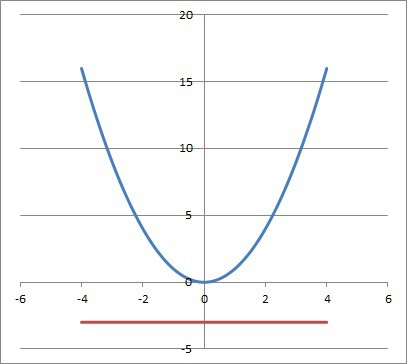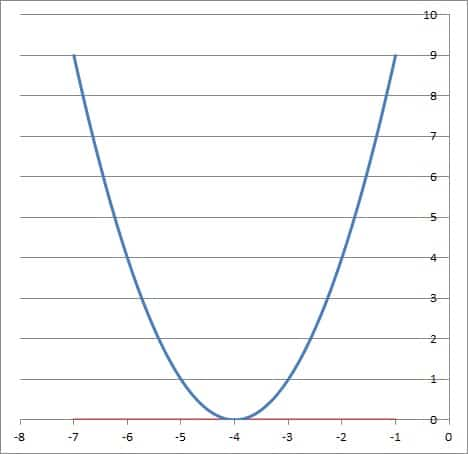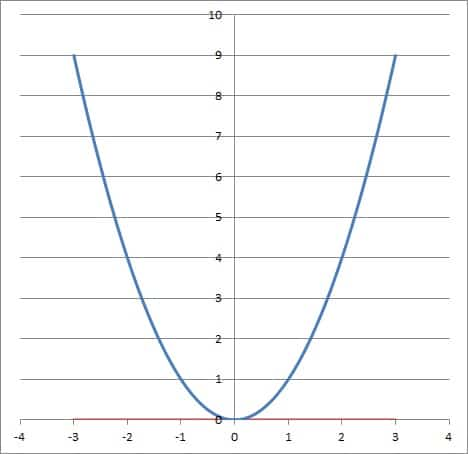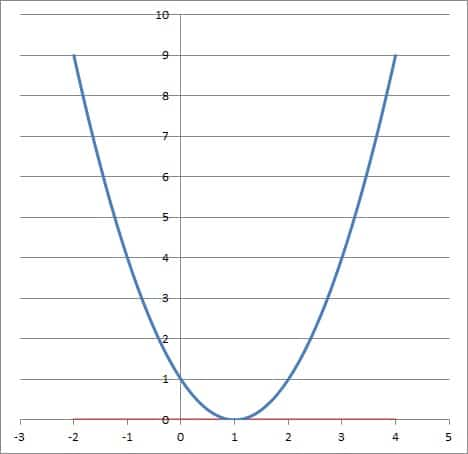# Can An Inequality Have No Solution? (3 Things To Know)

Inequalities are used to compare values and to express ranges or estimates in the case of uncertainty.  However, you might want to know whether an inequality has solutions and when it may fail to have them.

So, can an inequality have no solution?  An inequality can have no solution in several cases. Absolute value inequalities, compound inequalities, and quadratic inequalities can all have no solution in some cases. There are also cases where they can have only one solution (a single real number) or the set of all real numbers as solutions.

Of course, we can always find complex numbers to solve quadratic inequalities, but we will only consider real numbers for this discussion.

In this article, we’ll talk about when an inequality can have no solution and how it happens, along with some examples.  We’ll also talk about when an inequality has one solution or the set of real numbers as its solution set.

Let’s get started.

## Can An Inequality Have No Solution?

An inequality can have no solution, and there are several cases where this can happen, including:

Let’s take a closer look at each of these cases and some examples.  We’ll begin with absolute value inequalities.

### Absolute Value Inequalities With No Solution

Remember that an absolute value inequality contains the absolute value symbols.  You could absolute value inequalities like the following:

• |x| > 3
• |x – 2| <= 4
• |x| + |x – 1| > 0

For an absolute value inequality, the key thing to remember is that the absolute value symbol gives us a nonnegative number (the result is always positive or zero).

This means that |f(x)| >= 0 for any function f(x). You can learn more about absolute value in my article here.

With this in mind, let’s take a look at some examples of absolute value inequalities with no solution.

#### Example 1: Absolute Value Inequality With No Solution

Consider the following absolute value inequality:

• |x| < 0

It has no solution, because |x| >= 0.  That is, when we take the absolute value of any number x, we get zero (for x = 0) or a positive number (for x not equal to zero).

The absolute value of x, |x|, can never be negative, so it can never satisfy this inequality.  Thus, |x| < 0 has no solution.

#### Example 2: Absolute Value Inequality With No Solution

Consider the following absolute value inequality:

• |x| > |x| + 1

It has no solution, which we can see by subtracting |x| from both sides:

• |x| – |x| > |x| + 1 – |x|  [subtract |x| from both sides]
• 0 > 1  [|x| – |x| = 0 for all x]

This last inequality is never true, since 0 is less than 1.  So, regardless of which value of x we choose, we can never find one such that |x| > |x| + 1.  Thus, |x| > |x| + 1 has no solution.

### Compound Inequalities With No Solution

Remember that a compound inequality contains linking words such as “OR” & “AND”.

The “OR” word can give us a larger solution set, while “AND” can give us a smaller solution set.

For instance, x > 2 OR x < 3 gives us the set of all real numbers as the solution.  However, x > 2 AND x < 3 gives us the set (2, 3) or 2 < x < 3 as the solution set.

So, if we use “AND” to form a compound inequality, we can come up with one that has no solution.  Let’s see how that can happen.

#### Example 1: Compound Inequality With No Solution

Consider the following compound inequality:

• x > 0 AND x < 0

The “AND” wording means that both inequalities must be true at the same time.  This implies that we need a number x that is both positive (x > 0) and negative (x < 0).

Since there is no number that is both positive and negative, there is no solution.

Note: x = 0 is not a solution, since it satisfies neither inequality.  That is, zero is neither positive nor negative.

#### Example 2: Compound Inequality With No Solution

Consider the following compound inequality:

• x > 4 AND x < -3

The “AND” wording means that both inequalities must be true at the same time.  This implies that we need a number x that is both positive (x > 4) and negative (x < -3).

Since there is no number that is both positive and negative, there is no solution.

### Quadratic Inequalities With No Real Solution

Remember that a quadratic inequality is one with a quadratic expression on one side of the inequality symbol, such as:

• x2 > 0
• x2 + 5x > 0
• x2 + 6x + 9 <= 0

Note that we can always rearrange something like x2 + 4x < 5 so that it is in “standard” form, with a zero on one side: x2 + 4x – 5 < 0.

Let’s take a look at some quadratic inequalities with no real solutions.

#### Example 1: Quadratic Inequality With No Real Solution

• x2 <= -3

This inequality has no solution, and we can see this in several ways.  The first way is by reasoning about the signs (positive or negative).

Remember that x2 is always nonnegative for real x (that is, x2 >= 0).  So, we can never have x2 <= -3, since that would imply x2 is negative.

Another way to see that there is no solution is to graph both sides of the inequality to see that they never intersect.

The left side is a parabola y = x2, with its vertex at the origin (0, 0).  Note that this parabola never goes below the x-axis (since the vertex is its lowest point).

The right side is a horizontal line at y = -3.  This horizontal line is always below the parabola y = x2 (never above it).

Since the graph of y = x2 is always above the graph of y = -3, there is no solution to the inequality x2 <= -3.The graph of y = x2 (blue curve) is never below the horizontal line y = -3 (red line). So, the inequality has no solution.

#### Example 2: Quadratic Inequality With No Real Solution

• x2 + 8x + 16 < 0

This inequality has no solution, and we can see this in several ways.  The first way is by reasoning about the signs (positive or negative).

First, note that we can factor the left side as a perfect square trinomial to get:

• (x + 4)2 < 0

Remember that (x + 4)2 is always nonnegative for real x (that is, (x + 4)2 >= 0).  So, we can never have (x + 4)2 < 0, since that would imply (x – 4)2 is negative.

Another way to see that there is no solution is to graph both sides of the inequality to see that they never intersect.

The left side is a parabola y = (x + 4)2, with its vertex at the point (-4, 0).  Note that this parabola never goes below the x-axis (since the vertex is its lowest point).

The right side is a horizontal line at y = 0.  This horizontal line is always below the parabola y = (x – 4)2 (never above it).

Since the graph of y = (x + 4)2 is always above the graph of y = 0, there is no solution to the inequality (x + 4)2 < 0.The graph of y = x2 + 8x + 16 (blue curve) is never below the horizontal line y = 0 (red line). So, the inequality has no solution.

## Can An Inequality Have Only One Solution?

An inequality can have only one solution (that is, a single real value of x as the only solution).  This can happen with any of the above-mentioned inequalities: absolute, compound, and quadratic.

Let’s look at some examples, starting with absolute value inequalities with one solution.

### Absolute Value Inequalities With One Solution

An absolute value inequality can have only one solution in certain special cases.  Let’s see some examples.

#### Example 1: Absolute Value Inequality With One Solution

Consider the following absolute value inequality:

• |x| <= 0

It has one solution: x = 0.  This is because |x| >= 0.

That is, when we take the absolute value of any number x, we get zero (for x = 0) or a positive number (for x not equal to zero).

So the absolute value of x, |x|, can never be negative, so it can only satisfy this inequality when x = 0.  Thus, |x| < 0 has one solution: x = 0.

We can also write the solution set as {0}.

Note: we can also verify this by graphing the left and right sides of the inequality (y = |x| and y = 0) to see that the left side is never below the right side).

#### Example 2: Absolute Value Inequality With One Solution

Consider the following absolute value inequality:

• |x2 + 1| <= 1

It has one solution: x = 0.  We can prove this by using inequalities:

• x2 >= 0  [this is true for any real value of x]
• x2 + 1 >= 1  [add 1 to both sides of the inequality]
• |x2 + 1| >= |1|  [take the absolute value of both sides]
• |x2 + 1| >= 1

So, |x2 + 1| can never be less than 1.  However, |x2 + 1| can equal 1 when x = 0:

• |x2 + 1|
• =|02 + 1|
• =|0 + 1|
• =|1|
• =1

Thus, |x2 + 1| <= 1 has one solution: x = 0.

We can also write the solution set as {0}.

Note: we can also verify this by graphing the left and right sides of the inequality (y = |x2 + 1| and y = 1) to see that the left side is never below the right side).

### Compound Inequalities With One Solution

A compound inequality can also have only one solution in certain special cases.  Let’s see some examples.

#### Example 1: Compound Inequality With One Solution

Consider the following compound inequality:

• x >= 0 AND x <= 0

The “AND” wording means that both inequalities must be true at the same time.  This implies that we need a number x that is both nonnegative (x >= 0) and nonpositive (x <= 0).

There is only one number that is both nonpositive and nonnegative: x = 0.

We can also write the solution set as {0}.

#### Example 2: Compound Inequality With One Solution

Consider the following compound inequality:

• x >= 4  AND x <= 4

The “AND” wording means that both inequalities must be true at the same time.  This implies that we need a number x that is both at least 4 (x >= 4) and at most 4 (x <= 4).

There is only one number that satisfies both: x = 4.

We can also write the solution set as {4}.

### Quadratic Inequalities With One Solution

A quadratic inequality can have only one solution in certain special cases.  Let’s see some examples.

#### Example 1: Quadratic Inequality With One Solution

• x2 <= 0

This inequality has one solution: x = 0, and we can see this in several ways.  The first way is by reasoning about the signs (positive or negative).

Remember that x2 is always nonnegative for real x (that is, x2 >= 0).  So, we can never have x2 < 0, since that would imply x2 is negative.

The only option is x2 = 0, which happens when x = 0.

Another way to see that there is no solution is to graph both sides of the inequality to see that they never intersect.

If we graph y = x2 (the left side) and y = 0 (the right side), we see that the left side is never below the right side.  However, they do meet at the origin (the vertex of the parabola).The graph of y = x2 (blue curve) is equal to the horizontal line y = 0 (red line) when x = 0, but never below it. So, the inequality has one solution.

#### Example 2: Quadratic Inequality With One Solution

• x2 – 2x + 1 <= 0

This inequality has one solution: x = 1, and we can see this in several ways.  The first way is by reasoning about the signs (positive or negative).

Remember that x2 – 2x + 1 factors as a perfect square trinomial: (x – 1)2.  Since we are squaring, the expression (x – 1)2 is always nonnegative for real x (that is, (x – 1)2 >= 0).

So, we can never have (x – 1)2 < 0, since that would imply (x – 1)2 is negative.

The only option is (x – 1)2 = 0, which happens when x – 1 = 0, or x = 1.

Another way to see that there is no solution is to graph both sides of the inequality to see that they never intersect.

If we graph y = (x – 1)2 (the left side) and y = 0 (the right side), we see that the left side is never below the right side.  However, they do meet at the point (1, 0) (the vertex of the parabola).The graph of y = x2 -2x + 1 (blue curve) is equal to the horizontal line y = 0 (red line) when x = 1, but never below it. So, the inequality has one solution.

## Can An Inequality Have An Equal Sign?

An inequality can have an equal sign (>= or <=), as we saw in the earlier examples.  This just means that we include the endpoint as a solution of the inequality.

When we graph the inequality on a number line, we use a closed circle to indicate that the endpoint is included in the solution set.

For a strict inequality (with no equal sign), we use an open circle to indicate that the endpoint is not included in the solution set.

[image: graph inequalities on number line]

When we graph an inequality on coordinate axes (in 2 dimensions), we use a solid line to indicate that the line itself is included in the solution set.

For a strict inequality (with no equal sign), we use a dashed or dotted line to indicate that the line itself is not included in the solution set.

## Can An Inequality Equal Zero?

An inequality can equal zero in some cases.  This can happen if zero is in the solution set (such as x < 1) or if zero is one of the endpoints for an inequality with an equal sign (such as x >= 0).

## How Can The Solution Set For An Inequality Be All Real Numbers?

The solution set for an inequality can be all real numbers in several cases.  These should be familiar from our earlier discussion:

• Absolute Value Inequalities
• Compound Inequalities (with OR)
• Quadratic Inequalities (with an “x2” term)

### Absolute Value Inequalities With All Real Numbers As The Solution

An absolute value inequality can have all real numbers as the solution in some specific cases, as you can see below.

#### Example 1: Absolute Value Inequality With All Real Numbers As The Solution

Consider the following absolute value inequality:

• |x| >= 0

Every real number x is a solution, because |x| >= 0 for any real x.  That is, when we take the absolute value of any number x, we get zero (for x = 0) or a positive number (for x not equal to zero).

The absolute value of x, |x|, can never be negative, so it can never fail to satisfy this inequality.  Thus, |x| >= 0 has the set of all real numbers as its solution.

#### Example 2: Absolute Value Inequality With All Real Numbers As The Solution

Consider the following absolute value inequality:

• |x| >= -3

Every real number x is a solution, because |x| >= 0 for any real x, meaning that |x| >= -3 for all real x.

The absolute value of x, |x|, can never be negative, so it can never fail to satisfy this inequality.  Thus, |x| >= -3 has the set of all real numbers as its solution.

### Compound Inequalities With All Real Numbers As The Solution

A compound inequality can also have all real numbers as the solution in some specific cases, as you can see below.

#### Example 1: Compound Inequality With All Real Numbers As The Solution

Consider the following compound inequality:

• x >= 0 OR x <= 0

The “OR” wording means that one or both inequalities must be true.  This implies that we need a number x that is either nonnegative (x >= 0) or nonpositive (x <= 0).

Since all real numbers are either nonnegative or nonpositive, all real numbers x satisfy the compound inequality x >= 0 OR x <= 0.

#### Example 2: Compound Inequality With All Real Numbers As The Solution

Consider the following compound inequality:

• x >= -1 OR x <= 1

The “OR” wording means that one or both inequalities must be true.  This implies that we need a number x that is either greater than or equal to -1 (x >= -1) or less than or equal to -1 (x <= -1).

There is some overlap between these two sets, and when we take them both together, we get the entire set of real numbers.

Thus, all real numbers x satisfy the compound inequality x >= -1 OR x <= 1.

### Quadratic Inequalities With All Real Numbers As The Solution

A quadratic inequality can have all real numbers as the solution as well.  Here is an example.

#### Example: Quadratic Inequality With All Real Numbers As The Solution

• x2 >= 0

Every real number x is a solution to this inequality, and we can see this in several ways.  The first way is by reasoning about the signs (positive or negative).

Remember that x2 is always nonnegative for real x (that is, x2 >= 0).  So, any real number x we choose satisfies the inequality.

Another way to see that every real number is a solution is to graph both sides of the inequality to see that they always intersect.

When we graph y = x2 (left side) and y = 0 (right side), we can see that the left side is always above (or the same value as) the right side.  This means that x2 >= 0 for all values of x.The graph of y = x2 (blue curve) is equal to the horizontal line y = 0 (red line) when x = 0 and always above it. So, the inequality has all real numbers as its solution set.

## Conclusion

Now you know how an inequality can have no solution, one solution, or all real numbers as its solution set.  You also have some examples to help you see when these cases can occur.

You can learn about the uses of inequalities in everyday life here.